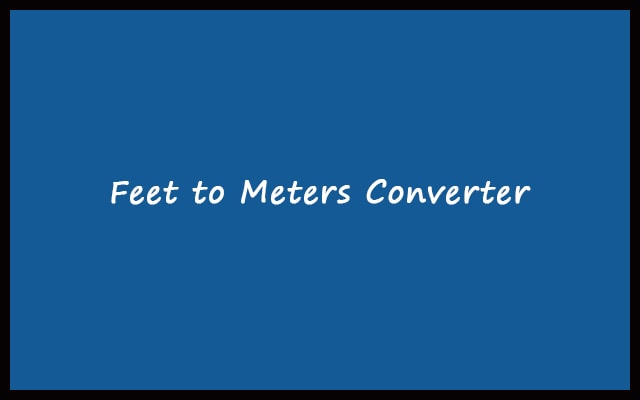# Feet to Meters – ft to m Converter Calculator Online

The feet and meters are two different units of measure. Both are using universally all over the world. In today’s world some company use feet in its measurement while some uses meter. In order to maintain the relationship, we need feet to meters converter.

Formula: 1 ft = 0.3048 feet
 Enter Feet: Meters Output: Meters + Centimeter Output: All Calculation:
RelatedIt can save your valuable time. Our feet to meters converter is absolutely free of cost. In our daily life, we frequently use the meter in the standard. Like for measuring distance in m. But sometimes we find measurements in feet. As we are human. Most of us have a strong connection with only one type of unit. For the sake of convenience, we use convertor. So, in that case, use feet to meters converter.

The length is recording in feet and meters. Instruments known as scale are using for it. Scales are also of different units. You select as per your preference. Both meter and feet can use to measure short as well as larger distances. Convert ft to m with our tool.

#### Feet Measurement System

The foot is the length of the imperial and US customary systems. The symbol of feet is “ft”. International yard and pound Agreement defined one foot as 0.0348 meters in 1959. One foot is equal to 12 inches and 3 feet. It is equal to 1 yard. In history, the foot was a part of many systems of units. Consisting of Roman, Chinese, and English as well as French. The United States is the only country that uses foot in preference to meter in its activities. In UK road signs use imperial units.

#### Meter Measurement System

The SI unit of length is meter. Also, it’s symbol is “m”. The meter is defining as path distance traveled by light in 1/299,792,458 of a second. The meter was first defined in 1793. It was defined as one ten-millionth of the distance from the equator to the North Pole. Firstly, in 1799 the meter was redefined. In 1960 meter was defined in terms of Kr-86 wavelengths. Lastly, in 1983 the current definition of meter was adopted and now in use. International Bureau of Weights and Measures has a prototype to define the meter. The prototype is a bar made up of 90% Platinum and 10% Iridium. One meter is the distance between two parallel lines on the bar.

## How to Convert Feet to Meters?

You have to understand the relationship between feet and meter. In order to start converting ft to m. The relationship between feet and meter is:
1 ft = 0.3048 m

So, in order to convert 3 feet into the meter. Just multiply 3 with 0.3048. Hence, 3 ft = 0.9144 m. Hence, the equation comes out to be.

##### meter = feet x 0.3048

Here,

d(m) stands for distance in m.
d(ft) stands for distance in feet.

Let’s have a look at some more examples. To clearly understand the process. The same mathematical process takes place inside the converter. Your input goes through the same process.

Example 1: Convert 96 feet to meters

We know that,

m = ft x 0.3048

Therefore, m = 96 x 0.3048 = 29.2608

Hence, As a result we get 96 ft = 29.2608 m

Example 2: 0.6 feet to meters

So, m = 0.6 x 0.3048 = 0.18288 m

Hence, 0.6 feet = 0.18288 meters

Example 3: Convert 5/2 feet to meters.

Here d(ft) = 5/2 ft = 2.5 ft

d(m) = 2.5 x 0.3048 = 0.762 m

Finally, 5/2 ft = 0.762 m

## Advantages of ft to m Converter

• While handling digits. It is very important to be accurate. Our converter shows 100% accuracy. It is because it works on pre-defined algorithms. Every input goes through the same process. Hence, no chances of error. Also, because there’s no human brain involved.
• Using the tool to convert ft to m. Saves your time & effort. It is very fast than manual calculation.

#### How to use ft to m converter?

2. Go to the ft to meters converter. Wait until it loads completely.
3. Enter the value of feet in the input box.
4. Press “Convert” button and as a result you can find the meter conversion value inside the below boxes.
5. Pressing “Reset” will clear all the values for a new conversion.

#### Feet to meters conversion table

Feet (ft) Meters (m)
0.01 ft 0.0030 m
0.1 ft 0.0305 m
1 ft 0.3048 m
2 ft 0.6096 m
3 ft 0.9144 m
4 ft 1.2192 m
5 ft 1.5240 m
6 ft 1.8288 m
7 ft 2.1336 m
8 ft 2.4384 m
9 ft 2.7432 m
10 ft 3.0480 m
20 ft 6.0960 m
30 ft 9.1440 m
40 ft 12.1920 m
50 ft 15.2400 m
60 ft 18.2880 m
70 ft 21.3360 m
80 ft 24.3840 m
90 ft 27.4320 m
100 ft 30.4800 m